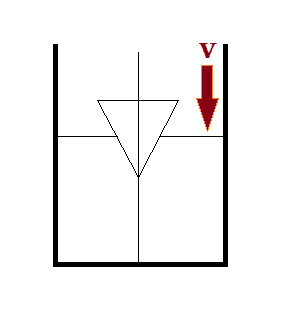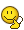# Rate of rise in water level

## Homework StatementA cylinder of radius ##{R}## is partially filled with water. There is an inverted cone of cone angle 90° and base radius ##{\frac{R}{2}}## which is falling in it with a constant speed ##v=30~cm/s##.
Find the rate of increase of water level (in ##cm/s##) when half the height of cone is immersed in water.

## Homework Equations

Let the height of the cone be h, and height of cylinder be H
Volume of cone ## = \frac{1}{3}\pi (\frac{R}{2})^2 h = \frac{1}{12} \pi R^2 h##
Volume of cylinder = ##\pi R^2 H##

## The Attempt at a Solution

Now for the cone ##\frac{dh}{dt}=v=30~cm/s##

Let the increase in cylinder's water level be ##dH## when ##dV## of cone's volume is immersed in it.
So
##dV = \pi R^2 dH##

⇒ ## \frac{dH}{dt} = \frac{\frac{dV}{dt}}{\pi R^2}##

⇒ ## \frac{dH}{dt} = \frac{\frac{d}{dt}(\frac{1}{12} \pi R^2 h)}{\pi R^2}##

⇒ ## \frac{dH}{dt} = \frac{\frac{1}{12} \pi R^2~\frac{dh}{dt}}{\pi R^2}##

⇒ ## \frac{dH}{dt} = \frac{1}{12} × 30 = 2.5~cm/s##

Thank you.

Homework Helper
Volume of the part of the cone that is immersed depends on h in a way that differs from what you use... The r of that part of the cone is dependent on h...

Vibhor
@BvU ,@Chestermiller ,do you agree with the given answer 2 cm/s ? I believe it is incorrect . It should be 1.875 cm/s .

2cm/s would have been correct if the cone was falling at the speed of 32 cm/s .

Last edited:
Mentor
@BvU ,@Chestermiller ,do you agree with the given answer 2 cm/s ? I believe it is incorrect . It should be 1.875 cm/s .

2cm/s would have been correct if the cone was falling at the speed of 32 cm/s .
I get 2 cm/s.

Let z be the distance between the tip of the cone and the bottom of the cylinder, and let h(z) be the distance between the water surface and the bottom of the cylinder. Then the amount of cone submerged is h - z, and the volume of cone submerged is $$V_s=\frac{\pi (h-z)^3}{3}$$. The total volume of water and cone below the water surface is ##\pi R^2h##, and this is also equal to the volume of water Vw plus the submerged volume of cone:

$$V_w+\frac{\pi (h-z)^3}{3}=\pi R^2h$$
Taking the time derivative of this equation gives:
$$(h-z)^2\left(\frac{dh}{dt}-\frac{dz}{dt}\right)=R^2\frac{dh}{dt}$$

•Vibhor
Vibhor
Hi ,

Let y be the distance between the tip of the cone and the water surface, and let h be the distance between the water surface and the bottom of the cylinder.

$$V_w =\pi R^2h - \frac{\pi y^3}{3}$$

Taking the time derivative of this equation gives: $$R^2\frac{dh}{dt} = y^2\frac{dy}{dt}$$ .

When ##y=\frac{R}{4}## , ##\frac{dh}{dt} = \frac{30}{16}##

What is the mistake ?

Mentor
Hi ,

Let y be the distance between the tip of the cone and the water surface, and let h be the distance between the water surface and the bottom of the cylinder.

$$V_w =\pi R^2h - \frac{\pi y^3}{3}$$

Taking the time derivative of this equation gives: $$R^2\frac{dh}{dt} = y^2\frac{dy}{dt}$$ .

When ##y=\frac{R}{4}## , ##\frac{dh}{dt} = \frac{30}{16}##

What is the mistake ?
The downward velocity of the cone is not dy/dt. It is (dy/dt)-(dh/dt).

•Vibhor
Vibhor
The downward velocity of the cone is not dy/dt. It is (dy/dt)-(dh/dt).Thanks a lot